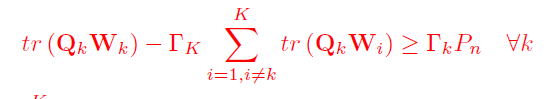# Problem with inequality constraints in CVXPY

Hi,
I have to use the following inequality of a SDP problem formulation in CVXPY:Q_k is a given matrix of size (N, N) with each entry being a complex value; {W_k} is the optimization variable, \gamma_k, P_n are given constant values.I used the following lines of code to write it in python using cvxpy:

def generate_h1(N_t):
# Generate h_1 as a N_t x 1 complex Gaussian vector
h_1 = np.sqrt(1/2)*np.random.randn(N_t, 1) + 1j * np.sqrt(1/2)*np.random.randn(N_t, 1)
return h_1

for k in range(K):
h_k = generate_h1(N_t)
h.append(h_k)

Q = [h_k * h_k.T.conj() for h_k in h]
Gamma = np.logspace(2, 20, K)
W = [cp.Variable((N_t,N_t), symmetric=True) for k in range(K)] # W_k

for k in range(K):
constraints.append(cp.trace(Q[k] @ W[k]) - np.real(Gamma[k])*cp.sum([cp.trace(Q[k] @ W[i]) for i in range(K) if i != k]) >= np.real(Gamma[k]) * P_n)
constraints.append(cp.sum([cp.trace(W[k]) for k in range(K)]) <= P_t)

the code gives the following error: ValueError: Inequality constraints cannot be complex. Could you please suggest a solution to this error?

Why should the LHS be real? It’s your model, in the sense that you are the one who found it in a paper or book (perhaps on the instructions of your advisor), so you should understand what the model is supposed to be.

If the LHS is supposed to be real, you obviously haven’t done something correctly, because the imaginary term is not just roundoff level. If the LHS is complex, should you be applying real(...) to it, or have separate inequalities for the real and imaginary parts? It’s “your” model so you should know what the mathematics is supposed to be .

In the future, if you are going to request help on the CVX forum, please post MATLAB/CVX code, not Python/CVXPY code.